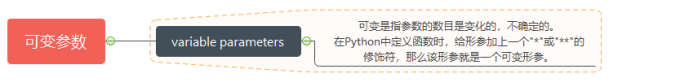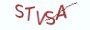Python基础教程

# 14.3.1 理解可变参数# 14.3.2 操作符*与**

(1) 使用*将实参收集为元组

```# __desc__ = 使用*操作符来定义一个可变形参

# 定义accumulate函数来实现累加
def accumulate(number, *numbers):
"""形参numbers是一个可变形参"""

sum_of_numbers = number

"""
(1) 使用*来修饰形参以后，Python会将该参数位置处开始的参数全部收集到
一个元组当中
(2) 元组就是使用*修饰的这个numbers形参
"""

# 在循环中遍历元组中的元素，并进行累加
for number in numbers:
sum_of_numbers += number

return sum_of_numbers

# 调用函数时，Python会将可变形参位置处开始的参数全部收集到一个元组中
sum_of_numbers = accumulate(1,2,3,4,5)
# Python会将实参2,3,4,5全部收集到元组numbers中
# sum_of_numbers的输出为15```

```# __desc__ = 使用*操作符来定义一个可变形参

# 定义accumulate函数来实现累加,将可变形参置于普通形参的左边
def accumulate(*numbers，number):
"""形参numbers是一个可变形参"""

sum_of_numbers = number

"""
(1) Python将形参numbers位置处开始的参数全部收集到
元组当中
(2)元组就是使用*修饰的这个numbers形参
"""

# 在循环中遍历元组中的元素，并进行累加
for number in numbers:
sum_of_numbers += number

return sum_of_numbers

# 在调用函数时，必须通过关键字参数的方式来调用accumulate函数
sum_of_numbers = accumulate(1,2,3,4,number = 5)

# 如果不通过关键字参数来调用，那么在执行时会抛出类型错误的异常```
(2) 使用**将实参收集为字典

```# __desc__ = 使用**操作符来定义一个可变形参

# 定义accumulate函数来实现累加,将可变形参置于普通形参的左边
def accumulate(**numbers):
"""形参numbers是一个可变形参"""

sum_of_numbers = 0

"""
(1)Python将形参numbers位置处开始的参数全部收集到字典当中
(2)字典就是使用**修饰的这个numbers形参
"""

# 在循环中遍历字典中的键名，并将键值进行累加
for _ in numbers:
sum_of_numbers += numbers[_]

return sum_of_numbers

# 在调用函数时，必须通过关键字参数的方式来调用accumulate函数
sum_of_numbers = accumulate(number1 = 5, number2=3)

# sum_of_numbers的输出为8```
(3) 混合使用"*"与"**"

```# __desc__ = 混合使用*与**操作符来对形参进行修饰

# 定义accumulate函数来实现累加
def accumulate(*numbers，**digits):

sum_of_numbers = 0
for _ in number:
sum_of_numbers += _

for _ in digits:
sum_of_numbers += digits[_]

return sum_of_numbers```
(4) *args与**kwargs
*args 与 **kwargs是可变形参名称的通用写法，前者用来将实参打包成一个元组类型，后者用来将实参打包成一个字典类型。同学们在实际定义函数的过程中，可以沿用这种通用的写法，也可以自行定义可变参数的名称。

```# __desc__ = 使用*args与**kwargs

# 定义accumulate函数来实现累加
def accumulate(*args，**kwargs):

sum_of_numbers = 0
for _ in args:
sum_of_numbers += _

for _ in kwargs:
sum_of_numbers += kwargs[_]

return sum_of_numbers```

# 14.3.3 对实参进行解包

```# __desc__ = 理解实参的解包

# 定义accumulate函数，将参数进行累加
def accumulate(number1, number2):
return number1 + number2

numbers = [1,2]

# 调用函数时使用*操作符对列表numbers进行修饰
sum_of_numbers = accumulate(*numbers)
# sum_of_numbers的输出为3```

(1) 使用*对可迭代对象进行解包

```# __desc__ = 使用*序列结构进行解包

# 定义accumulate函数，将参数进行累加
def accumulate(param1, param2):
return param1 + param2

# 1.对字符串进行解包
chars = "ab"
result = accumulate(*chars)
# result的输出为"ab"

# 2.对列表进行解包
numbers = [1,2]
result = accumulate(*numbers)
# result的输出为3

# 3.对元组进行解包
numbers = (5,5)
result = accumulate(*numbers)
# result的输出为10

# 4.对集合进行解包
numbers = {3,-3}
result = accumulate(*numbers)
# result的输出为0```
(2) 使用**对字典进行解包

```# __desc__ = 使用**字典进行解包

# 定义accumulate函数，将参数进行累加
def accumulate(x, y):
return x + y

numbers = {"x": 1, "y": 99}

result = accumulate(**numbers)
# result的输出为100```

```# __desc__ = 使用**字典进行解包

# 定义accumulate函数，将参数进行累加
def accumulate(small, large):
return small + large

numbers = {"small": 1, "large": 99, "median": 33}
result = accumulate(**numbers)```

# 14.3.4 知识要点

(1) 定义函数时，使用*与**操作符来定义可变参数，在调用函数时，使用*与**操作符来对参数进行解包。
(2) 使用*操作符定义可变参数时，Python会将该形参位置处开始的实参收集到元组中。
(3) 使用**操作符定义可变参数时，Python会将该形参位置处开始的实参收集到字典中。

# 14.3.5 最具实力的小班培训

`(1) Python后端工程师高薪就业班，月薪11K-18K，免费领取课程大纲(2) Python爬虫工程师高薪就业班，年薪十五万，免费领取课程大纲(3) Java后端开发工程师高薪就业班，月薪11K-20K, 免费领取课程大纲(4) Python大数据分析，量化投资就业班，月薪12K-25K,免费领取课程大纲`## 注册账号